# Control flow in Python: break and continue

2022-01-31 23:41:01 PI dada

official account ： Youer cottage
author ：Peter
edit ：Peter

Hello everyone , I am a Peter~

I've introduced Python Medium for、if、 Assignment and other related statements , This paper introduces Python Two control flows in ：break and continue

First , From the literal meaning of English words , We can simply understand the two ：

• break, Break means , In the code, it is expressed as interrupting the operation of the following code
• continue, Go on , It is reflected in the code to continue the operation of the following code

secondly , What we need to understand is that both Out of the loop The role of , Can be used in for Circulation and while In circulation , But the scope of action is different .

This article mainly explains two main knowledge points ：

• break and continue sentence
• The impact of indentation on code blocks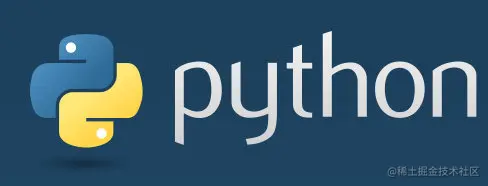## range function

In the following examples range(m,n,s) The function represents taking the value of the specified value interval as the iterator ：

• m： The starting position , Integers ; The default from the 0 Start , You can get it. m; Omission
• n： Termination position , Integers , There has to be , Can't get n; Don't omit
• s： step , The length of each retrieval ; Positive but negative

Here's an example ：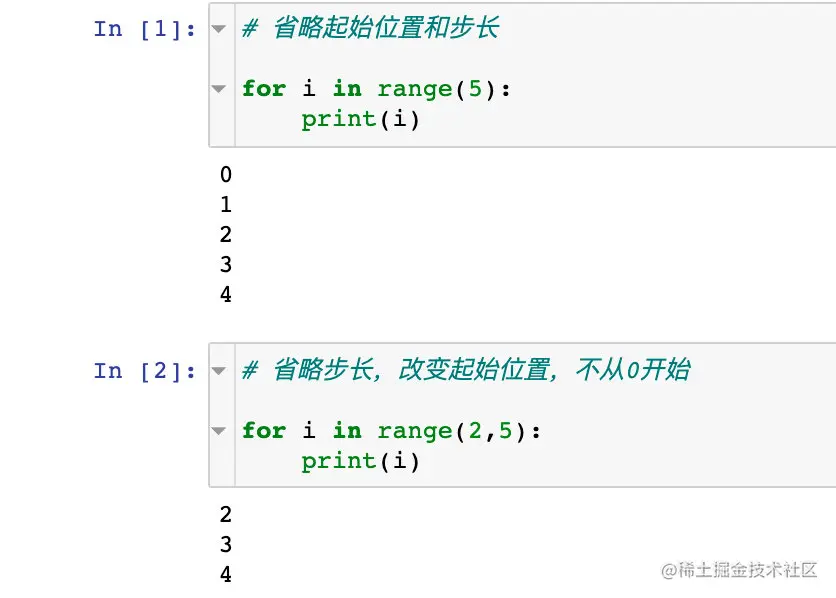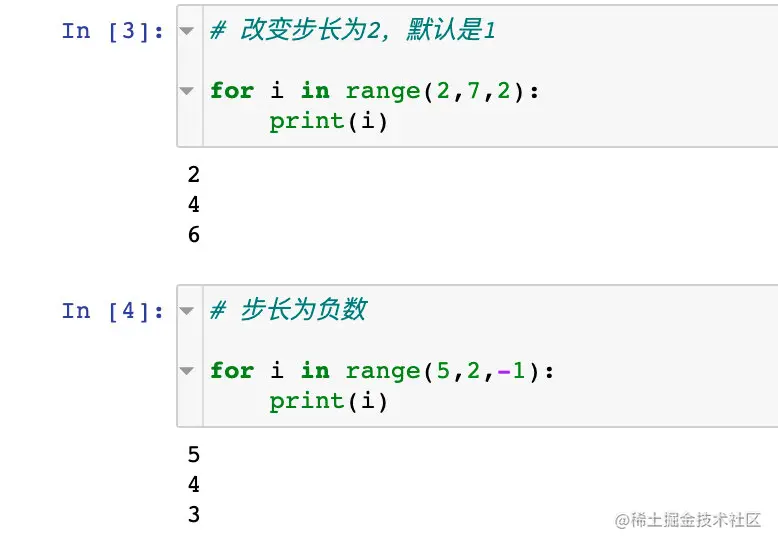## monolayer for loop -break

Example 1：

``````for i in range(8):  # i=0,1,2....7
if i == 5:  #  When i be equal to 5 When it comes to execution break operation
break
print(i)

#  result
0
1
2
3
4
Copy code ``````

How to explain the above results ？

• When i=0, Get into if, It's not equal to 5, Don't execute break, direct print, Now output 0
• Same thing ,i=1,2,3,4 It's all the same thing
• When i=5 Get into if In the sentence , Find out i=5, perform break, Out of the whole cycle , hinder i=6、7 It won't be executed

Look at examples 2（ Just not indented ）：

``````for i in range(8):   # i=0,1,2....7
if i == 5:
break
print(i)

#  result
5
Copy code ``````

This example illustrates the impact of indentation on code execution ：

• i=0 Conduct if, It's not equal to 5, Don't execute break, Enter the next cycle, i.e i=1
• Same thing , When i=1、2、3、4 Conduct if, It's not equal to 5, Don't execute break
• When i=5 Get into if When , It just equals 5, perform break Statement jumps out of the entire loop , here i just 5, Print out print(i) Namely 5

Example 3：

The results of this example are explained in the same way as the example 1, Just to range The iterator of the function is replaced by a string ：

``````for i in "python":
if i == "o":
break
print(i)

#  result
p
y
t
h
Copy code ``````

These three break Relevant examples illustrate ： When you meet with satisfaction break Conditions , Will jump out of the whole for loop ; Of course , This is only for single-layer cycles .

## monolayer for loop -continue

Example 4：

``````for i in range(8):  # i=0,1,2....7
if i == 5:
continue
print(i)

#  result
0
1
2
3
4
6
7
Copy code ``````

Explain the above results ：

• When i=0 Get into if loop , It's not equal to 5, Don't execute continue, perform print(i), Print 0; Into the next loop
• When i=1、2、3、4 Get into if In the cycle , It's the same effect , And execute print(i) The sentence of
• When i=5 Get into if loop , just i be equal to 5, perform continue, Jump out of the print(i), That is, don't print 5; Go directly from the beginning to the next cycle
• When i=6、7 Just like i=0、1、2、3、4 The explanation is the same

If print The statement is not indented ？ Look at examples 5：

``````for i in range(8):
if i == 5:
continue
print(i)

#  result
7
Copy code ``````

From the example above 5 The results can be seen , The last time i Is equal to 7; When our print When the statement is not indented , Only when for The statement will not be executed until it is completely executed , So wait until the last i=7 Will print .

Example 6：

``````for i in [" Chinese language and literature "," mathematics "," English "]:
if i != " mathematics ":
continue
print(i)

#  result
mathematics
Copy code ``````

Explain the above results ：

• i by “ Chinese language and literature ”, First entry if sentence ,i It's not math , perform continue, Not execute print; Into the next loop
• The second time i The value is “ mathematics ”, Get into if in , dissatisfaction if Conditions , So don't execute continue, perform print, Print out “ mathematics ”
• hinder “ English ” Satisfy if Conditions , direct continue Jumped out

above 3 individual continue Relevant examples illustrate ： When satisfied continue The condition of the statement , It will jump out of the current do not execute this time , But it does not affect the execution of the following statements

## Gauss sums

Example 7：

``````sum = 0  #  The initial value of and

for i in range(1,101):
if i % 2 == 0:  # i Divide 2 The remainder is 0, namely i It's even
break
sum += i  # sum = sum + i
print(sum)

#  result
1
Copy code ``````

Explain the above results ：

• When i=1 Get into if, dissatisfaction if Conditions , perform sum += i The sentence of , here sum Turned into 1,i Turned into 2 Into the next loop
• When i=2 Get into if, Satisfy the even condition , perform break, Straight out of the loop , hinder sum+i No more execution
• print(sum) It's actually the first step sum value 1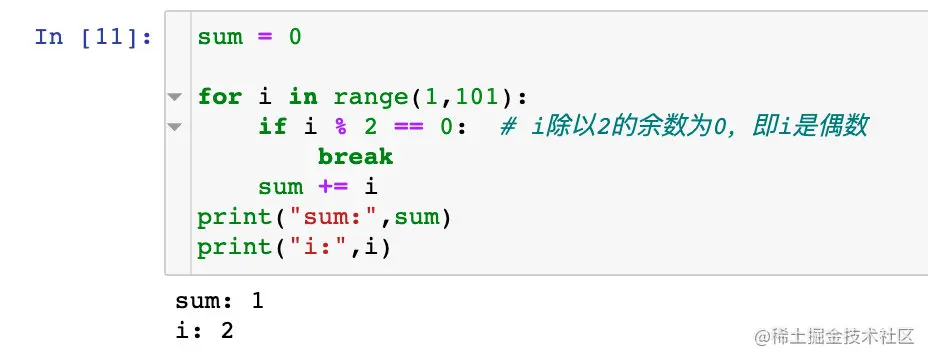Example 9：

``````sum = 0

for i in range(1,101):   # i from 1 To 100
if i % 2 == 0:    # i Divide 2 The remainder is 0, namely i It's even
continue
sum += i
print(sum)

#  result
2500
Copy code ``````

Explain the above results ：

• When i=1 Get into if loop , dissatisfaction if Conditions （i It's odd ）, perform `sum += i`,sum become 1,i become 2 Into the next loop
• When i=2 Get into if loop , Just enough if Conditions （i For the even ）, perform continue, Do not execute the following `sum += 1`,i become 3 Into the next loop
• When i=3 Get into if loop , dissatisfaction if Conditions （i It's odd ）, perform `sum += i`,sum become 1+3=4,i become 4 Into the next loop
• When i=4 Get into if loop , Just enough if Conditions （i For the even ）, perform continue, Do not execute the following `sum += 1`,i become 5 Into the next loop

Before passing 4 Step we found ： Only when i The addition operation will only be performed when it is an odd number , So it's actually 1 To 100 The sum of odd numbers of , just 2500

## double for loop -break

Example 10：

``````for i in range(3):  # i：0、1、2
for j in range(4):  # j：0、1、2、3
if j > i:
break
print((i,j))  #  and if alignment

#  result
(0, 0)
(1, 0)
(1, 1)
(2, 0)
(2, 1)
(2, 2)
Copy code ``````

Explain the above results ：

The above code contains two layers for loop , from i=0 Enter the first layer of circulation ：

i j Is it satisfactory? if perform break Whether to carry out print
0 0 no yes ,（0,0）
0 1 yes no
0 2 Skipped
0 3 Skipped
1 0 no yes ,（1,0）
1 1 no yes ,（1,1）
1 2 Skipped
1 3 Skipped
2 0 no yes ,（2,0）
2 1 no yes ,（2,1）
2 2 no yes ,（2,2）
2 3 Skipped

This is the execution and interpretation of the whole process ~

Example 11：

``````for i in range(3):    # i=0,1,2
for j in range(4):   # j=0,1,2,3
if j > i:
break
print((i,j))  #  And the second for alignment

#  result
(0, 1)
(1, 2)
(2, 3)
Copy code ``````

Explain the above results ：

The difference between this example and the above one is ：print And the second for alignment , That is, only when the second for Only after all operations are completed will print

i j Is it satisfactory? if perform break Whether to carry out print
0 0 no no
0 1 yes yes ,（0,1）
0 2 Skipped
0 3 Skipped
1 0 no no
1 1 no no
1 2 yes yes ,（1,2）
1 3 Skipped
2 0 no no
2 1 no no
2 2 no no
2 3 yes yes ,（2,3）

in other words ： When two-layer cycle ,break Will jump out of the innermost layer （ by break lately ） Of for loop

Example 12：

``````for i in range(3):
for j in range(4):
if j > i:
break
print((i,j))

#  result
(2, 3)
Copy code ``````

The explanation of the above results is the same as that of the first two , Until the last time （2,3） Meet the conditions break The condition will execute print.

## double for loop -continue

Example 13：

``````for i in range(3):  # i=0,1,2
for j in range(4):  # j=0,1,2,3
if j > i:
continue
print((i,j))

#  result
(0, 0)
(1, 0)
(1, 1)
(2, 0)
(2, 1)
(2, 2)
Copy code ``````

Explain the above results ：

i j Is it satisfactory? if perform continue Whether to carry out print
0 0 no yes ,（0,0）
0 1 yes , Jump out of
0 2 yes , Jump out of
0 3 yes , Jump out of
1 0 no yes ,（1,0）
1 1 no yes ,（1,1）
1 2 yes , Jump out of
1 3 yes , Jump out of
2 0 no yes ,（2,0）
2 1 no yes ,（2,1）
2 2 no yes ,（2,2）
2 3 yes , Jump out of

Example 14：

``````for i in range(3):
for j in range(4):
if j > i:
continue
print((i,j))

#  result
(0, 3)
(1, 3)
(2, 3)
Copy code ``````

Explain the above results ：

print The code of the statement is indented , Only when the second floor for All done , Will print

i j Is it satisfactory? if perform continue Whether to carry out print
0 0 no no
0 1 yes , Jump out of no
0 2 yes , Jump out of no
0 3 yes , Jump out of yes ,（0,3）
1 0 no no
1 1 no no
1 2 yes , Jump out of no
1 3 yes , Jump out of yes ,（1,3）
2 0 no no
2 1 no no
2 2 no no
2 3 yes , Jump out of yes ,（2,3）

Example 15：

``````for i in range(3):
for j in range(4):
if j > i:
continue
print((i,j))

#  Take the last value
(2, 3)
Copy code ``````

Summarize the essential differences between the two ：break Is used to terminate The whole cycle ; and continue It's about jumping out This cycle , It does not affect the execution of the next cycle .

## Artifact website

Finally, I give you a classic artifact website ：pythontutor.com/visualize.h…

Let's take this code as an example ：

``````for i in range(3):
for j in range(4):
if j > i:
continue
print((i,j))

#  result
(0, 3)
(1, 3)
(2, 3)
Copy code ``````

1、 Write code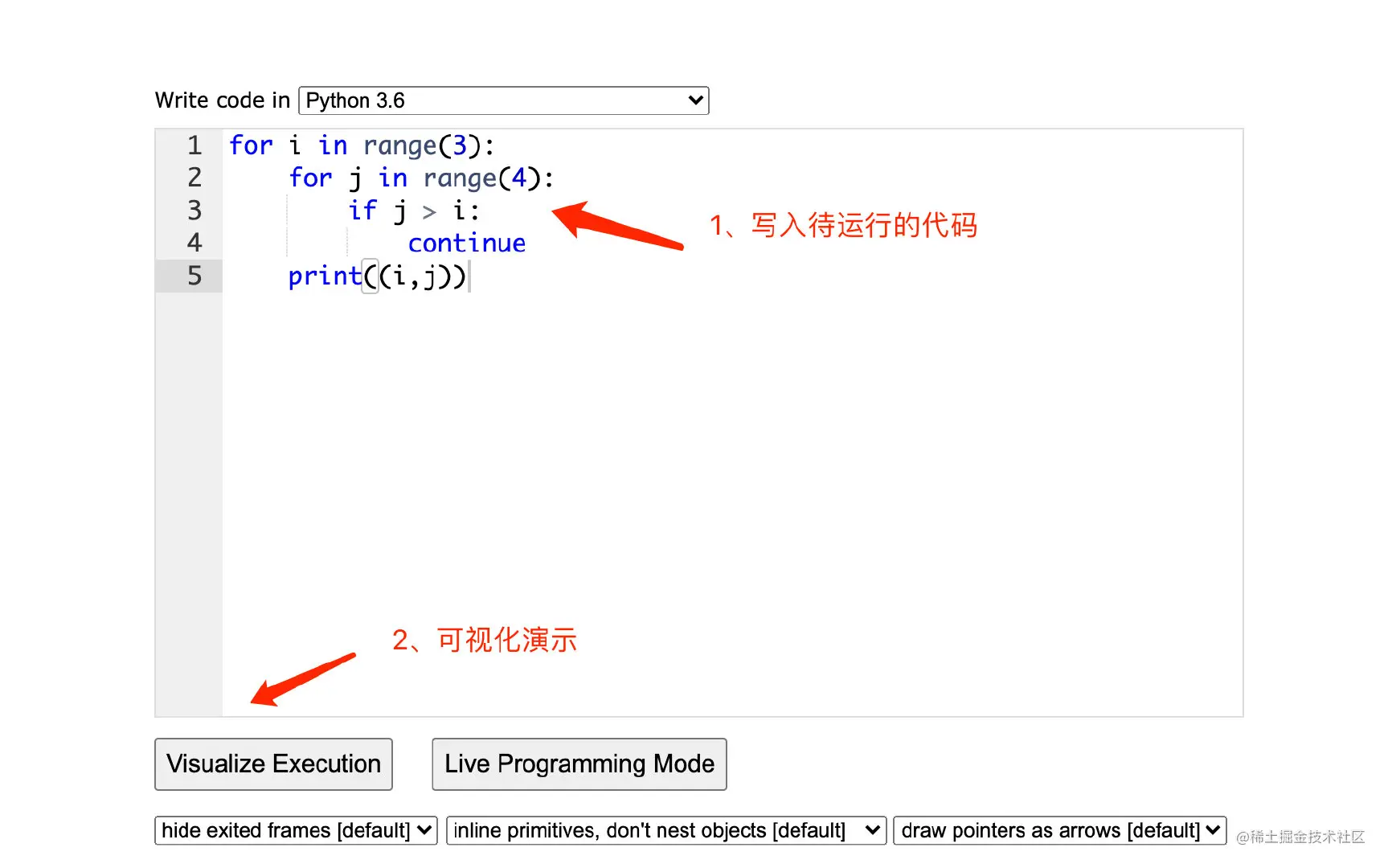2、 Demonstration process ：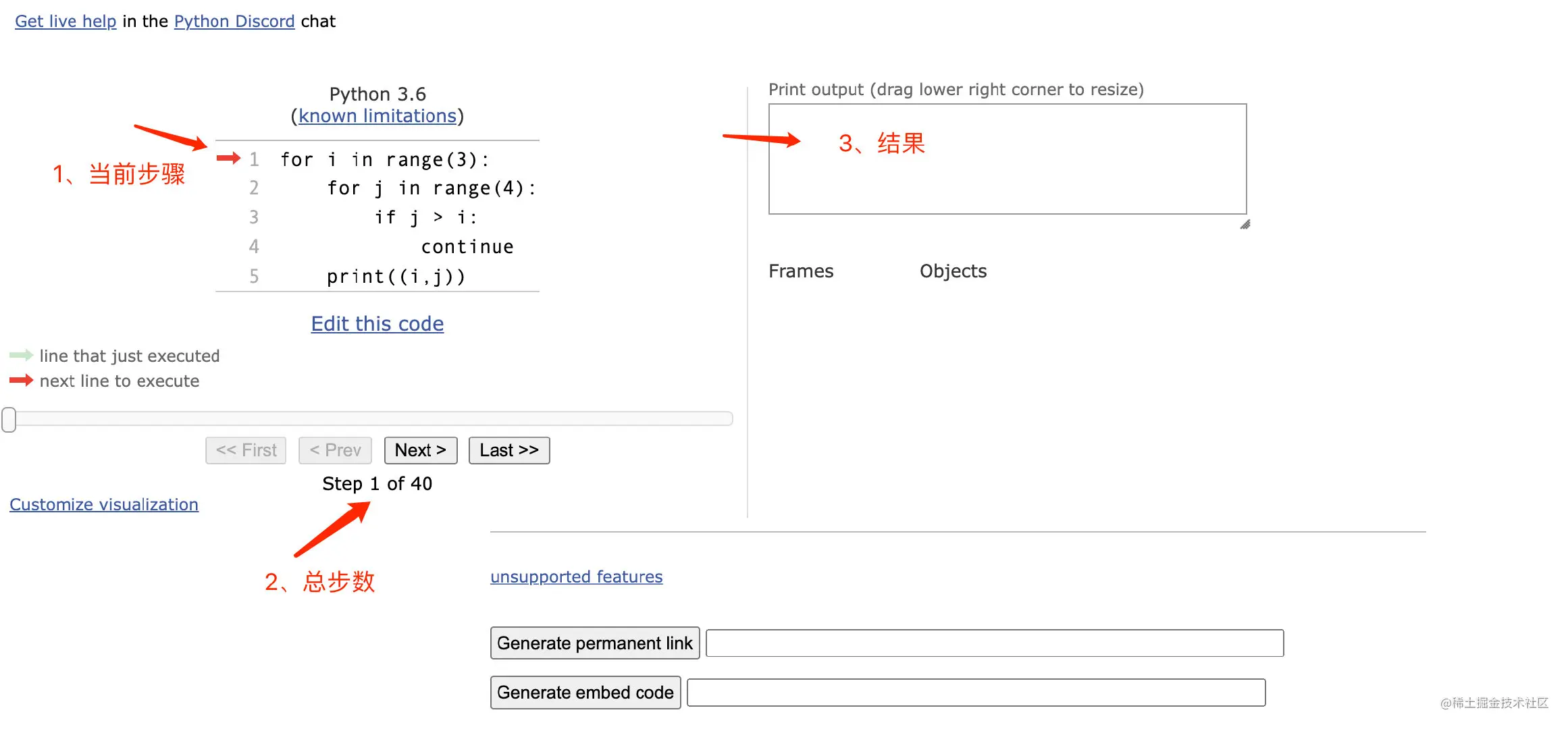3、 The specific process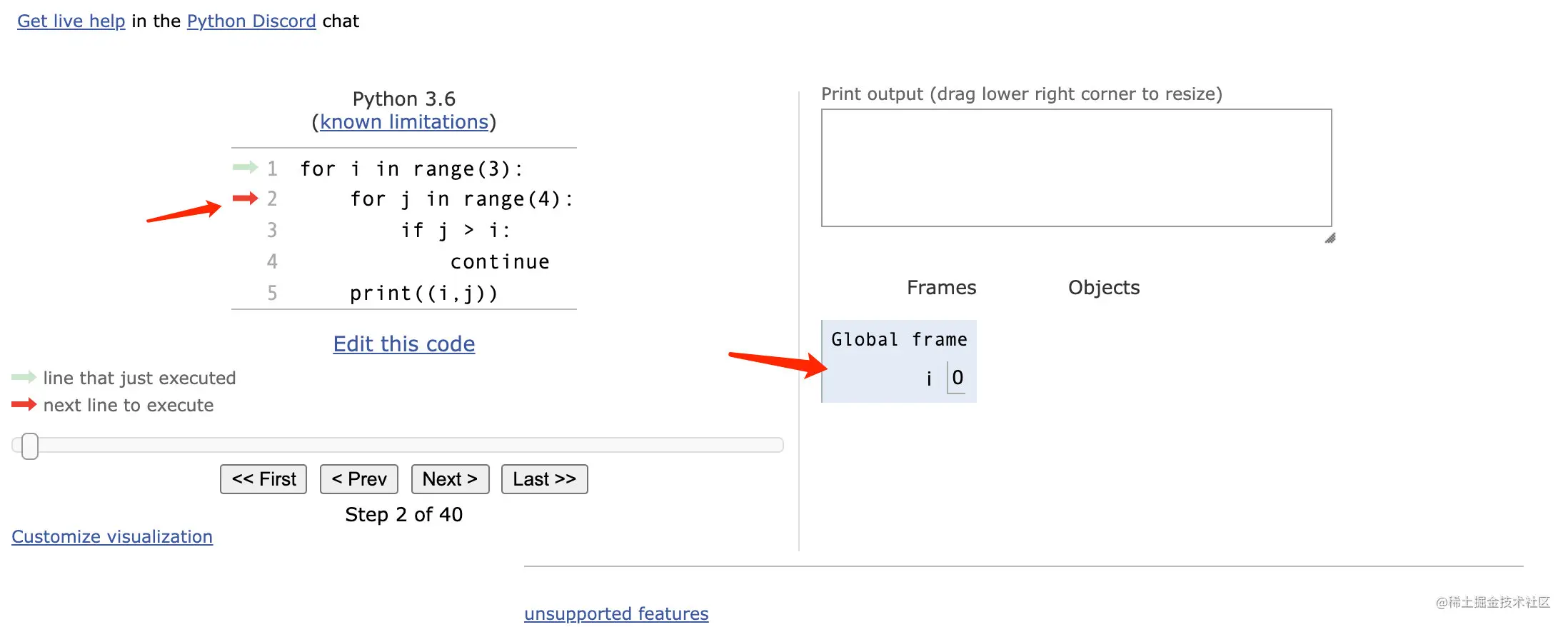When we run to the 15 step , The first result ：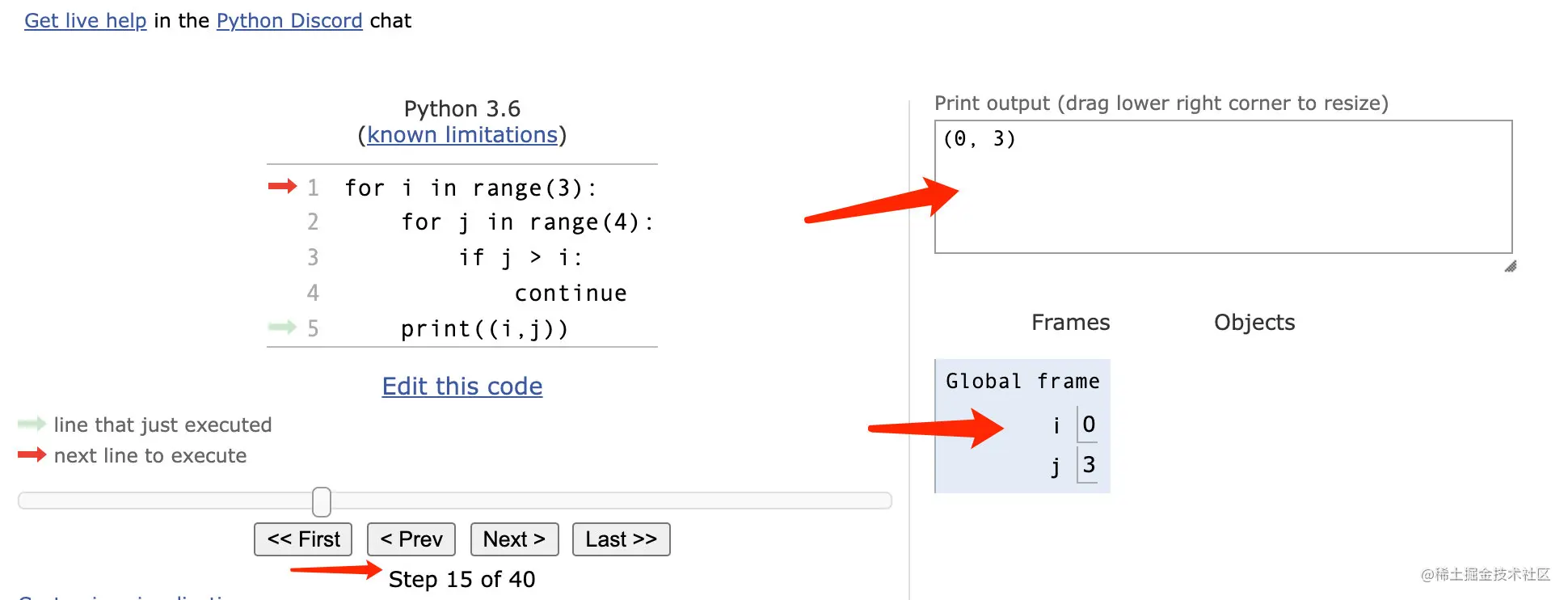40 All steps are finished , We see the result we want ：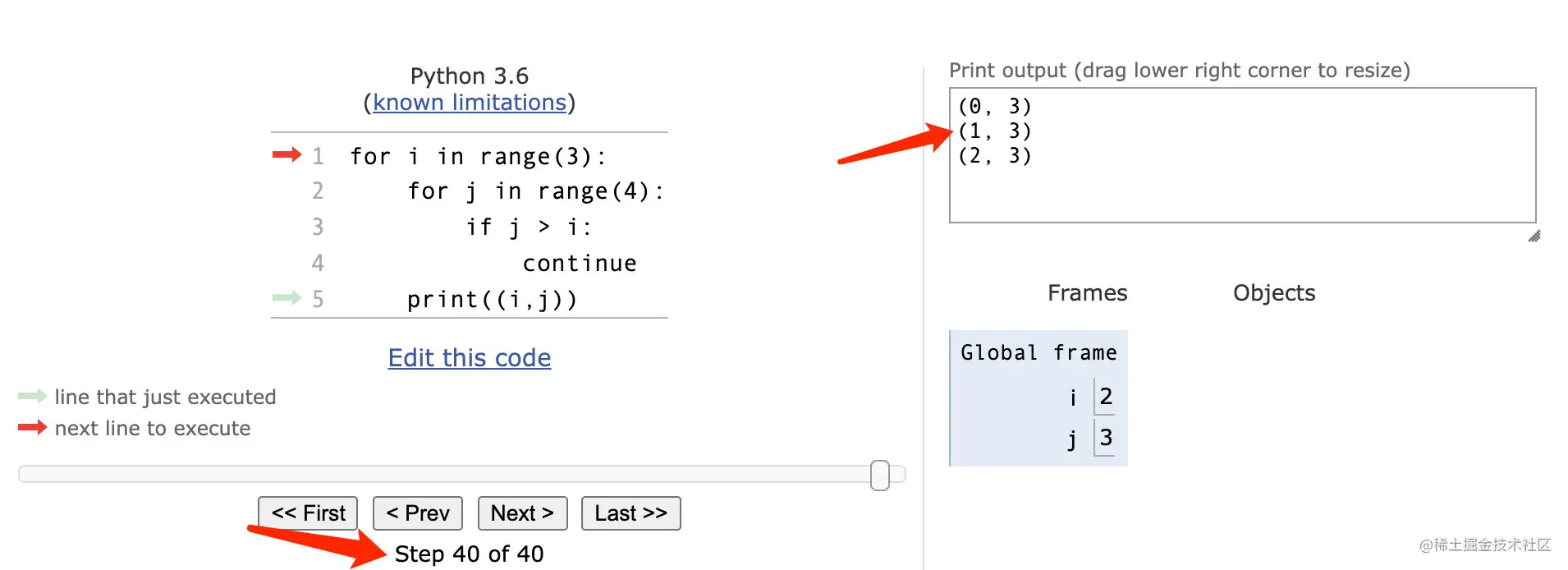Interested friends can go to this website to see ~